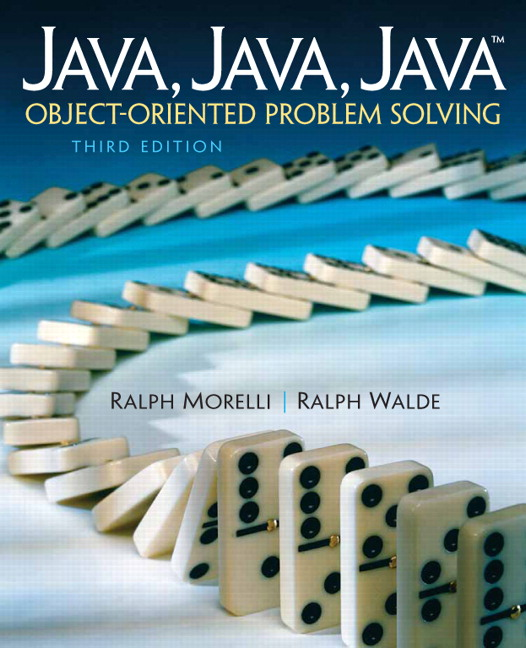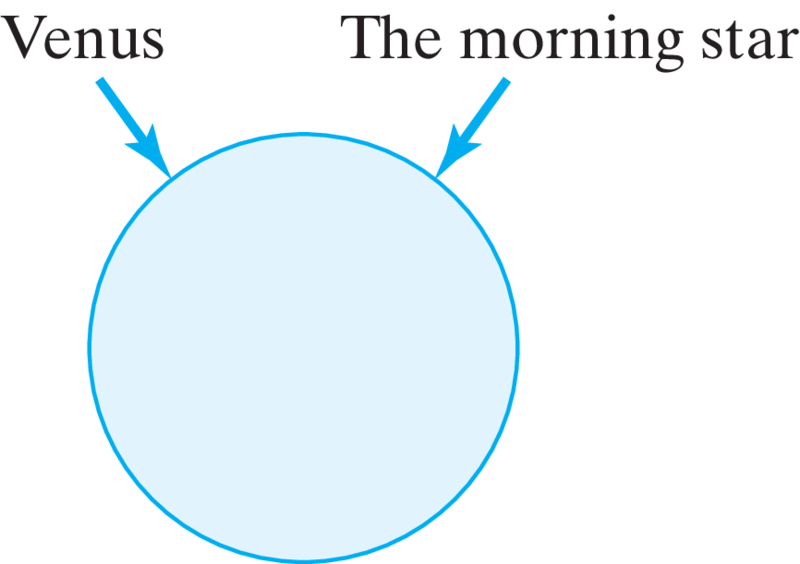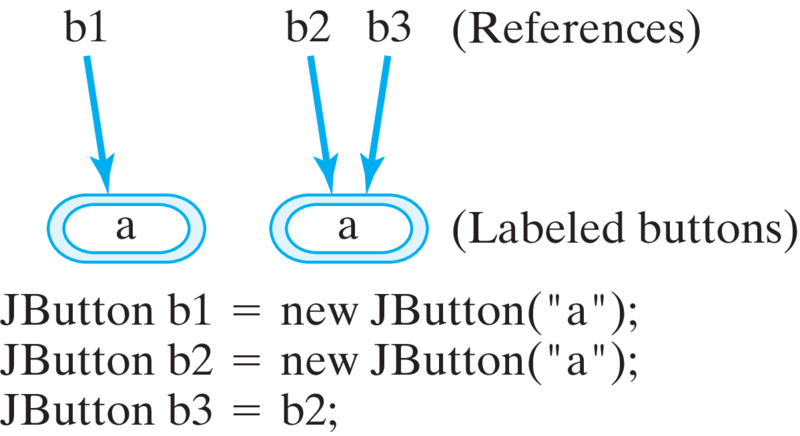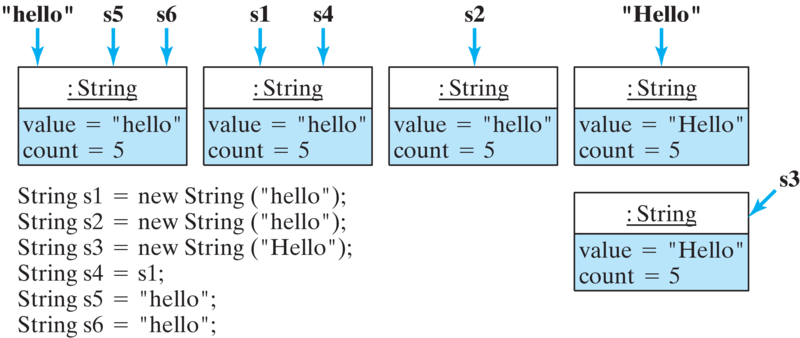# Java, Java, Java: Object-Oriented Problem Solving, 2022E

## Section7.9Comparing Strings

Comparing strings is another important task. For example, when a word processor performs a search and replace operation, it needs to identify strings in the text that match the target string.

### Subsection7.9.1Lexicographic Order

Strings are compared according to their lexicographic order — that is, the order of their characters. For the letters of the alphabet, lexicographic order just means alphabetical order. Thus, a comes before b and d comes after c. The string “hello” comes before “jello” because h comes before j in the alphabet.
For Java and other programming languages, the definition of lexicographic order is extended to cover all the characters that make up the character set. We know, for example, that in Java’s Unicode character set the uppercase letters come before the lowercase letters (Table 5.13). So, the letter H comes before the letter h and the letter Z comes before the letter a.
Lexicographic order can be extended to include strings of characters. Thus, “Hello” precedes “hello” in lexicographic order because its first letter, H, precedes the first letter, h, in “hello.” Similarly, the string “Zero” comes before “aardvark,” because Z comes before a.
To determine lexicographic order for strings, we must perform a character-by-character comparison, starting at the first character and proceeding left to right. As an example, the following strings are arranged in lexicographic order:
"" "!" "0" "A" "Andy" "Z" "Zero" "a" "an" "and" "andy" "candy" "zero"

We can define lexicographic order for strings as follows:
Perhaps a more precise way to define lexicographic order is to define a Java method:
This method does a character-by-character comparison of the two strings, proceeding left to right, starting at the first character in both strings. Its for loop uses a counting bound, which starts at k equal to zero and counts up to the length of the shorter string. This is an important point in designing this algorithm. If you don’t stop iterating when you get past the last character in a string, your program will generate a StringIndexOutOfBounds exception. To prevent this error, we need to use the shorter length as the loop bound.
Note that the loop will terminate early if it finds that the respective characters from s1 and s2 are unequal. In that case, s1 precedes s2 if s1’s kth character precedes s2’s. If the loop terminates normally, that means that all the characters compared were equal. In that case, the shorter string precedes the longer. For example, if the two strings were “alpha” and “alphabet,” then the method would return true, because “alpha” is shorter than “alphabet.”

#### ExercisesSelf-Study Exercises

##### 1.Lexicographic Order.
Arrange the following strings in lexicographic order: alpha zero bath Alpha Zero a A
##### 2.StringFollows.
Using the precedes() method 7.9.2 as a guide, define and test a follows method. It should return true if and only if s1 follows s2 in lexicographic order.

### Subsection7.9.2Object Identity Versus Object Equality

Java provides several methods for comparing String s:
public boolean equals(Object anObject); // Overrides Object.equals()
public boolean equalsIgnoreCase(String  anotherString);
public int compareTo(String  anotherString);

The first comparison method, equals(), overrides the Object.equals() method. Two String s are equal if they have the exact same letters in the exact same order. Thus, for the following declarations,
String s1 = "hello";
String s2 = "Hello";

s1.equals(s2) is false, but s1.equals("hello") is true.
You have to be careful when using Java’s equals() method. According to the default definition of equals(), defined in the Object class, “equals” means “identical.” Two objects are equal only if their names are references to the same object.
This is like the old story of the morning star and the evening star, which were thought to be different objects before it was discovered that both were just the planet Venus. After the discovery, it was clear that “the morning star” and “the evening star” and “Venus” were just three different references to one and the same object (Figure 7.9.3).Figure 7.9.3. Venus is the morning star and the evening str.
We can create an analogous situation in Java by using the following JButton definitions:
JButton b1 = new Button("a");
JButton b2 = new Button("a");
JButton b3 = b2;

Given these three declarations, b1.equals(b2) and b1.equals(b3) would be false, but b2.equals(b3) would be true because b2 and b3 are just two names for the same object (Figure 7.9.4). So, in this case, “equals” really means “identical.”Figure 7.9.4. For most objects, equality means identity. JButtons b2 and b3 are identical (and, hence, equal), but JButtons b1 and b2 are not identical (and, hence, unequal).
Moreover, in Java, when it is used to compare two objects, the equality operator (==) is interpreted in the same way as the default Object.equals() method. So, it really means object identity. Thus, b1 == b2 would be false, because b1 and b2 are different objects, but b2 == b3 would be true because b2 and b3 refer to the same object.
These points are illustrated in the program shown in Listing 7.9.5. This program uses methods isEquals() and isIdentical() to perform the comparisons and print the results.
This program will produce the following output:
java.awt.Button[button0,0,0,0x0,invalid,label=a]
does NOT equal java.awt.Button[button1,0,0,0x0,invalid,label=b]
java.awt.Button[button0,0,0,0x0,invalid,label=a]
does NOT equal java.awt.Button[button1,0,0,0x0,invalid,label=b]
java.awt.Button[button1,0,0,0x0,invalid,label=b]
equals java.awt.Button[button1,0,0,0x0,invalid,label=b]
java.awt.Button[button0,0,0,0x0,invalid,label=a]
is NOT identical to java.awt.Button[button1,0,0,0x0,invalid,label=b]
java.awt.Button[button0,0,0,0x0,invalid,label=a]
is NOT identical to java.awt.Button[button1,0,0,0x0,invalid,label=b]
java.awt.Button[button1,0,0,0x0,invalid,label=b]
is identical to java.awt.Button[button1,0,0,0x0,invalid,label=b]


### Subsection7.9.3String Identity Versus String Equality

In comparing Java String objects, we must be careful to distinguish between object identity and string equality. Thus, consider the following declarations:
String s1 = new String("hello");
String s2 = new String("hello");
String s3 = new String("Hello");
String s4 = s1;            // s1 and s4 are now identical
String s5 = "hello";
String s6 = "hello";

which create the situation shown in Figure 7.9.6.Figure 7.9.6. For String objects, equality and identity are different. Two distinct (nonidentical) String objects are equal if they store the same string value. So s1, s2, s4, s5, and s6 are equal. Strings s1 and s4 are identical, and so are strings s5 and s6.
Given these declarations, we would get the following results if we compare the equality of the Strings:
s1.equals(s2) ==> true  s1.equalsIgnoreCase(s3)  ==> true
s1.equals(s3) ==> false s1.equals(s5)            ==> true
s1.equals(s4) ==> true  s1.equals(s6)            ==> true

and the following results if we compare their identity:
s1 == s2  ==> false            s1 == s3  ==> false
s1 == s4  ==> true             s1 == s5  ==> false
s5 == s6  ==> true

The only true identities among these Strings are s1 and s4, and s5 and s6. In the case of s5 and s6, both are just references to the literal string, “hello”, as we described in Section 7.2.1. The program in Listing 7.9.7 illustrates these points.

#### ExercisesSelf-Study Exercises

##### 1.String equality vs identity.
Evaluate the following expressions as true or false based on these String declarations,
String s1 = "java", s2 = "java", s3 = "Java";
String s4 = new String(s2);
String s5 = new String("java");

1. s1 == s2
2. s1.equals(s2)
3. s1 == s3
4. s1.equals(s3)
5. s2 == s3
Hint.
Review this program Listing 7.9.7) and its discussion.
##### 2.String equality vs identity Part 2.
Evaluate the following expressions as true or false based on these String declarations,
String s1 = "java", s2 = "java", s3 = "Java";
String s4 = new String(s2);
String s5 = new String("java");

1. s2.equals(s4)
2. s2 == s4
3. s1 == s5
4. s4 == s5
Hint.
Review this program Listing 7.9.7) and its discussion.
##### 3.String Play.
Use indexOf() and substring() methods to manipulate the strings s1 and s2 as described. Use System.out.println() to test your code.